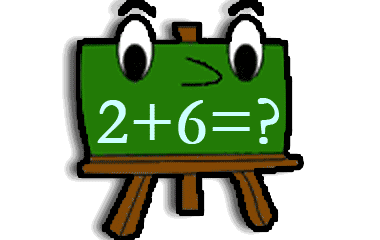1
One term is 1
2
One term is 2
3
One term is 3
4
One term is 4
5
One term is 5
6
One term is 6
7
One term is 7
8
One term is 8
9
One term is 9
10
One term is 10
11
One term is 11
12
One term is 12
13
One term is 13
14
One term is 14
15
One term is 15
16
One term is 16
17
One term is 17
18
One term is 18
19
One term is 19
20
One term is 20
21
One term is 21-25
22
One term is 26-30
23
One term is 31-35
24
One term is 36-40
25
One term is 41-45
26
One term is 46-50
27
One term is 51-55
28
One term is 56-60
29
One term is 61-65
30
One term is 66-70
31
One term is 71-75
32
One term is 76-80
33
One term is 81-85
34
One term is 86-90
35
One term is 91-100

## Recent activities in this game

Someone had a problem at level 1. One term is 1 .
1 hour ago after 9 seconds of playing.
Someone had a problem at level 1. One term is 1 .
1 hour ago after 7 seconds of playing.
Someone got all questions correct at level 6. One term is 6 .
1 hour ago after 13 minutes of playing.
Someone had a problem at level 12. One term is 12 .
2 hours ago after 7 seconds of playing.
Someone had a problem at level 1. One term is 1 .
2 hours ago after 5 seconds of playing.
Someone got all questions correct at level 3. One term is 3 .
3 hours ago after 5 minutes of playing.
Someone had a problem at level 1. One term is 1 .
3 hours ago after 3 seconds of playing.
Someone got all questions correct at level 2. One term is 2 .
3 hours ago after 4 minutes of playing.
Someone got all questions correct at level 2. One term is 2 .
3 hours ago after 3 minutes of playing.
Someone got all questions correct at level 2. One term is 2 .
4 hours ago after 4 minutes of playing.

Add the two terms and figure out the sum for all the numbers! Fun game to practice addition for students in school.Choose a game level among the buttons above. This will give you up to ten tasks that you must solve by adding together the two numbers and find the sum. Can you solve all the additions in a level, you will get one knowledge points and the button for that level turns green.

### Addition in the language of mathematics

Addition belongs to the four arithmetic modes and the correct expression is to add two numbers. Some might say Sum two numbers, which also is a mathematically correct way of expressing an addition.

Two terms add up to the sum:
 4 + 7 = 11 term + term = sum

Below is the addition table which helps the student to learn how to add by selecting column and row and see the sum of the two numbers.
+012345678910
0012345678910
11234567891011
223456789101112
3345678910111213
44567891011121314
556789101112131415
6678910111213141516
77891011121314151617
889101112131415161718
9910111213141516171819
101011121314151617181920

### Calculation of knowledge points

Each completed game level gives 1 knowledge point in Addition. The maximum number of points (35 knowledge points) is achieved when you pass all 35 levels. You'll get a bronze medal when you complete a level 2 times and a silver medal after 5 completed rounds. A gold medal will be received after 10 completed rounds. In Addition, the maximum number of collectable gold medals is 35. You only earn knowledge points for levels that has not been cleared before. A cleared level has a green background above. Even when you have completed a level, you can continue practicing at that level, but it gives you no more knowledge points.

Math
Math
Math
Math
Math
Math

Subject
Math
Target group
Practices
Game creator
Updated
2019-09-26
Published online
2011-11-11
Unique id
1
Saved by
10-20 users
Operating system
Browser
Price
Free of charge

3.8
Total 348
5
209
4
28
3
22
2
20
1
69

### Report

If you find a spelling mistake or something else that should be corrected, feel free to contact the game creator or us at Helpful Games.

Write a comment below to contact the game creator or